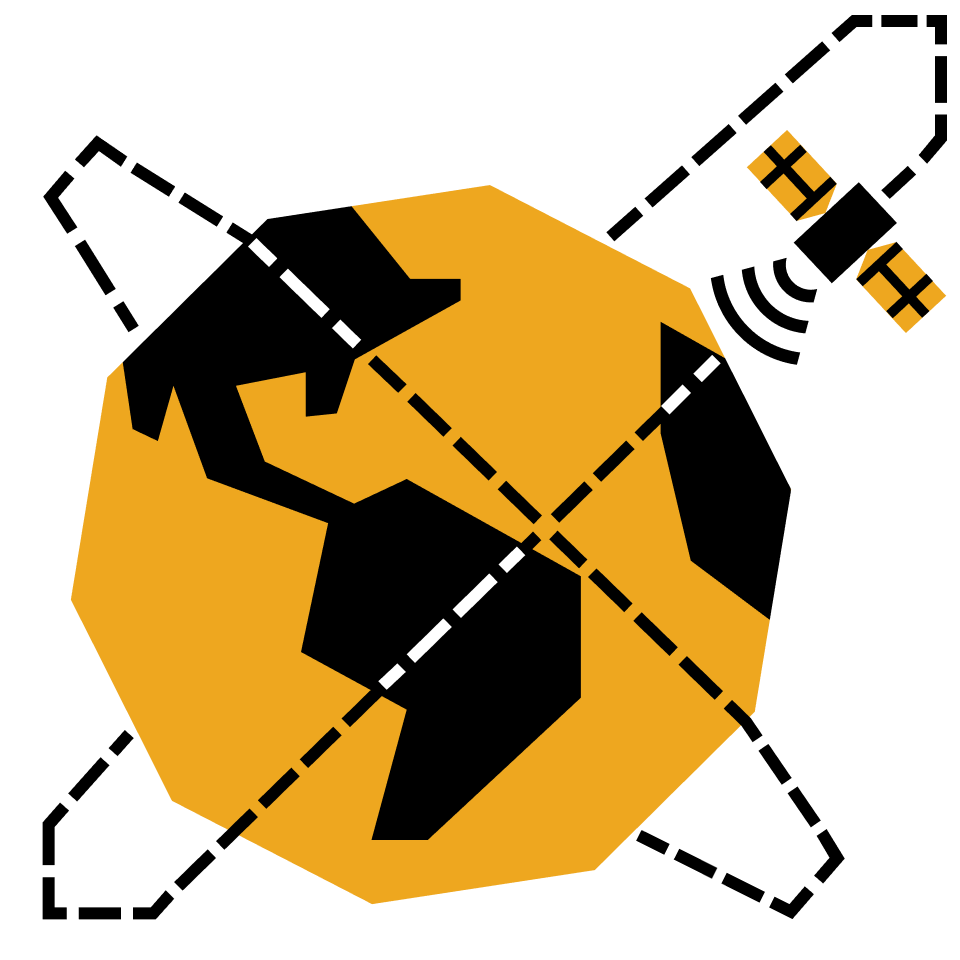Science · Level 3

## 3.3 Gravitational Physics

Explore Newton's law of gravity and unpack its universe of consequences.

Explore: Mass Dependence

Explore: Distance Dependence

Apply: Heavenly Orbits

The Strength of Gravity

Cavendish's Experiment

Atmospheric Thickness

Escape Velocity

Ocean Tides

Gravitational Redshift

Gravity is Acceleration

Gravitational Energy

Gravitational Pendulum

Full Field of a Spherical Shell

Gauss' Law

Hunting for Resources

What if Earth was Flat?

Why Planets are Smooth

Circular Orbits

The Two-body Problem

Equations of Orbital Motion

Rotational Symmetry

Orbits in a Gravity Well

Bound Orbits

Elliptical Orbits

Hohmann Transfer Orbits

Orbital Periods

### Course description

Here we lay out Newton's law of gravity and crack open the universe of consequences that spring from it. On the journey we'll come to understand planetary phenomena like tides and atmospheres, the rich geometry of heavenly bodies and their motion, and the engineering considerations of space travel. By the end of this course, you’ll be able to code simulations to explore applied problems using `Python`, derive pieces of general relativity, and understand where Newton’s law starts to buckle.

### Topics covered

• Angular Momentum
• Black Holes
• Equivalence Principle
• Gauss’ Law
• Gravitational Fields
• Gravitational Lensing
• Kepler’s Laws
• Orbital Mechanics
• Slingshot Effect
• Tides

### Prerequisites and next steps

You’ll need an understanding of mathematics and physics at the level of Classical Mechanics.

### Prerequisites

• Classical Mechanics
• Calculus in a Nutshell

Up next

Science · Level 4

### 4.1 Solar Energy

Learn the physics of energy harvesting from our most renewable source, the Sun.